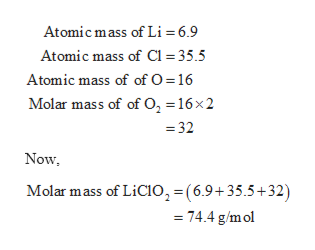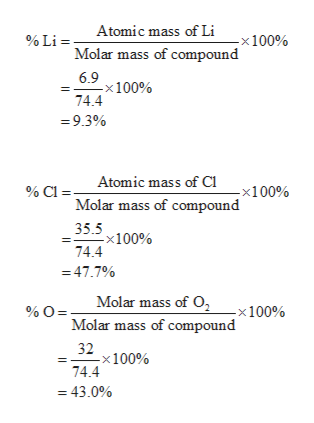Calculate the percent composition by mass of each element in LiClO2

Question

Calculate the percent composition by mass of each element in LiClO2

Step 1

The molar mass of the compound LiClO2 can be calculated as follows:help_outlineImage TranscriptioncloseAtomic mass of Li = 6.9 Atomic mass of C1 35.5 Atomic mass of of O = 16 Molar mass of of 0, = 16x2 = 32 Now Molar mass of LiCIO2 (6.9+35.5+32) = 74.4 g/mol fullscreen
Step 2

The percent composition by mass of each element in Li...help_outlineImage TranscriptioncloseAtomic mass of Li 100% % Li = Molar mass of compound 6.9 -x100% 74.4 =9.3% Atomic mass of Ci % Cl=. -x100% Molar mass of compound 35.5 -x100% 74.4 47.7% Molar mass of O %O= -x 100% Molar mass of compound 32 :100% 74.4 =43.0% fullscreen

Want to see the full answer?

See Solution

Want to see this answer and more?

Our solutions are written by experts, many with advanced degrees, and available 24/7

See Solution
Tagged in

Chemistry